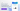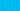Pricing# What is time value of money?

Written by

Would you rather have \$75 now, or \$100 in three months? This question is at the heart of the time value of money concept. In some cases, immediate access gives money extra value. Keep reading to learn more about the time value of money and how it’s calculated.

## Understanding the time value of money

The time value of money is a simple concept used in accounting and investing. This idea claims that money in the present holds more value than the same sum received in the future.

There are two primary reasons for this.

1. If you have a sum of money right now, it can be invested and grown into a larger future sum.

2. There is some risk involved with future monetary predictions, for example, if the payer goes bankrupt.

Time value of money is very similar to net present value (NPV).

## What is time value of money with an example?

To look more closely at what is time value of money with an example, let’s explore the following scenario.

Imagine that you’re completing some work, and your employer gives you two payment options.

1. In the first option, you can receive \$5000, right now.

2. In the second option, you will receive \$5500, but not until one year in the future.

Many of us would automatically take the \$5000 right now, but time value of money goes deeper than that to analyse which option is more valuable.

To start with, you must look at the money’s growth. A sum of \$5500 is 110% of \$5000, which gives you a 10% return on the original sum. The first factor to think about with any time value of money calculator is rate of return. Could you make more than 10% on the initial \$5000 by investing it yourself? If so, you should take the money right now.

Otherwise, it would be more financially beneficial to take the \$5500 in one year, provided the employer is trustworthy, and there is no chance of their business going bankrupt.

## Time value of money and inflation

Another factor to weigh into the equation is inflation. Inflation cuts into the value of money over time, perhaps best illustrated by commodity prices like petrol or food prices. If someone gave you a voucher worth \$100 for petrol, you would receive very different amounts of petrol in 1995 and 2020, for example.

This is why inflation, along with purchasing power, is another factor that weighs into the actual time value of money. When an investment’s real return is calculated, the rate of inflation is always subtracted from the return percentage. If inflation is higher than the investment return rate, you would be losing money in purchasing power.

Let’s take the example given above. Imagine you’ve opted for the \$5500 payment. This gives you a 10% return on the initial sum. However, the rate of inflation this coming year is 15%, which means you lose 5% of your purchasing power. Due to inflation, the current \$5000 is more valuable than the future \$5500.

## Features of time value of money

To sum up, the factors that account for money’s value over time include:

• Rate of return

• Inflation percentage

Understanding the time value of money is vital for businesses who need to make decisions about when to purchase equipment, develop products, or establish credit terms.

## Time value of money formula

You can also plug some of these features of time value of money into a formula:

FV = PV x [ 1 + (i / n) ] (n x t)

Where:

• FV = Future value of money

• PV = Present value of money

• i = interest rate

• n = number of compounding periods per year

• t = number of years

This formula isn’t perfect because there is always some degree of the unknown when it comes to future predictions. However, it gives you some idea of future value to use when weighing any investment.

## We can help

GoCardless helps you automate payment collection, cutting down on the amount of admin your team needs to deal with when chasing invoices. Find out how GoCardless can help you with ad hoc payments or recurring payments.